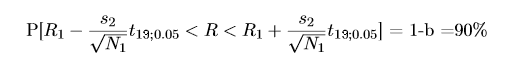# Combining standard deviations

We have two groups measuring the same resistors, the nominal value is unknown. Group 1 is slower and because of that they did not calcute the s1 empirical standard deviation.
• Group 1: N1=500 , R1=6903 , s1=unknown
• Group 2: N2=20 , R2=6880 , s2=168.3
,where N1,N2 is number of measurements, R1,R2 is average of the measured values and s1,s2 empirical standard deviations

We have to give the confidence interval for the nominal value of the resistance at confidence level p=90% and prove that this is the right way to calculate it.

Somewhere i found an answer, but i dont really understand why is it correct and also i need to prove that its right.

The solution was this: "Even if we dont know s1, we can use estimator R1, because its more accurate than R2 (because of the larger amount of information). However we can only use s2 empirical standard dev. and the confidence interval should be calculated by student´s-t distribution with degree of freedom N2-1=19"The s2 is divided by root square of N1 however the student´s distribution has degree of freedom N2. Can someone explain me, why is this correct, why can we combine the two measurements this way or better show me the deduction?

## Answers and Replies

RUber
Homework Helper
Degrees of freedom are for ##s_2##, so it makes sense that you would use 19.
There is an underlying assumption that the variances in the samples are equal. This seems fair, since the groups are measuring the same resistors.
I would assume that you could also add in the information from group 2 into your mean data as well if you wanted to.
## \overline{R} = \frac{500(6903)+20(6880)}{520} ##
## P[ \overline{R} - \frac{s_2}{\sqrt{N_2+N_1}}t_{19,0.05} < R < \overline{R} + \frac{s_2}{\sqrt{N_2+N_1}}t_{19,0.05} ] = 90\%##

I doubt the intervals would be much different either way. In any case, I would normally argue for including more data whenever possible.

Last edited:
RUber
Homework Helper
To add a little more clarity to the matter:
The 90% confidence interval for any one observation based on the observed standard deviation and mean would be:
## P[ \overline{R} - s_2 t_{19,0.05} < R < \overline{R} + s_2 t_{19,0.05} ] = 90\%##
However, the mean of the data will vary much less...reduced by a factor of ##\sqrt{N}## where N is the number of observations used to determine the mean.
The T distribution tends toward the normal Z distribution for large samples, but for small samples is a fatter curve, which makes for wider confidence intervals than a true normal. This is to account for uncertainty in the estimator for the population standard deviation--in this case ##s_2##. The degrees of freedom in the T distribution are n-1 where n is the number of observations used to estimate ##s_2##.
Does that make sense?

Yes, thank you! Is there any way I can prove that: $$\frac{\bar{R}-R}{\frac{s_2}{\sqrt{N_1+N_2}}}=t_{19,0.05}$$?

RUber
Homework Helper
It is based on the assumption that N1 and N2 are pulled from the same population. If they are, then there is no reason not to add the 20 trials from N2 into the overall observed mean unless you can speculate as to some reason they might have yielded slightly lower values.
So then if you assume they are one population, then you get an estimator for the mean, which is ##\overline{R}## based off of N1+N2 =N trials.
Now the question is: what about the variance of the mean?
We can only estimate based on what we know. Using our best estimate for the variance, ##s_2^2##, we again assume that all the resistors are from the same population and use the T distribution.

I am not sure what you are asking for in terms of a proof...

RUber
Homework Helper
Okay, I think I found a solid proof.
I came across the following--refer to Montgomery et al Introduction to Linear Regression Analysis 5th ed. p. 576:

" If ##Z ## ~ ## N(0,1)## and ##V## ~##\chi^2##, and Z and V are independent, then ##\frac{Z}{\sqrt{V/\nu}} ## ~## t_\nu ## "

Given that ##\frac{\overline{R} - \mu }{\frac{\sigma}{\sqrt{520}}}## is normally distributed with mean 0 and stdev = 1, that will serve as Z.
Also, ##\sqrt{\frac{s_2^2}{\sigma^2}}## ~ ##\sqrt{\frac{\chi_{19}^2}{19} }##, this will serve as ##\sqrt{V/\nu}##.
Therefore, ## \frac{ \frac{\overline{R} - \mu }{\frac{\sigma}{\sqrt{520}}} } {\sqrt{\frac{s_2^2}{\sigma^2}} }
= \frac{\overline{R} - \mu }{\frac{\sigma}{\sqrt{520}}} \frac{1}{ \frac{s_2}{\sigma}}
= \frac{\overline{R} - \mu }{\frac{s_2}{\sqrt{520}}}## ~ ##t_{19}##.

Again, the fundamental assumption is that the samples came from the same population, so they can be combined. If there is reason to question this assumption, then there may also be reason to assume that you should question that the variances would be the same between samples.